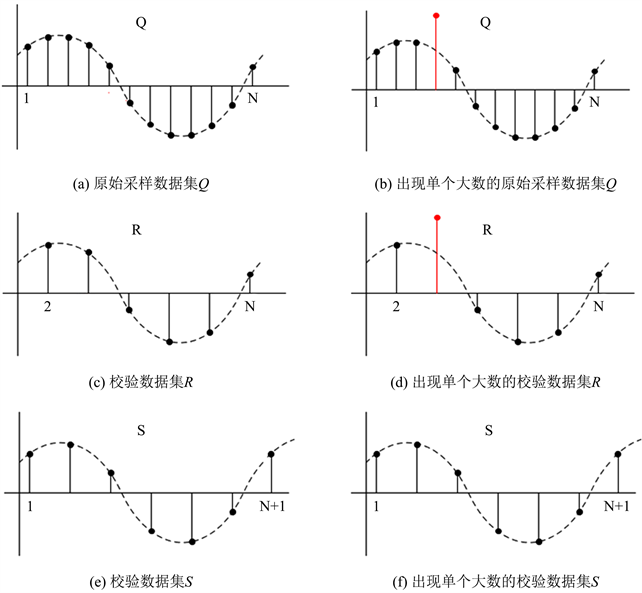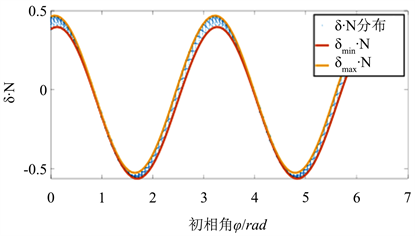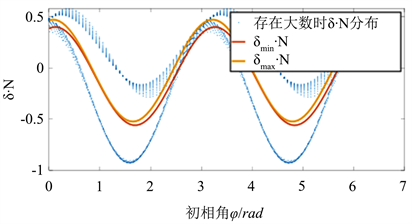#### 期刊菜单

Interference Identification Method of AC Signal
DOI: 10.12677/SG.2021.116040, PDF , HTML, XML, 下载: 255  浏览: 405

Abstract: Full-cycle Fourier algorithm is usually used to analyze discrete AC sampling data in microcomputer protection. When there is interference data in AC signal, it will affect phasor analysis and get wrong system measurement results. In this paper, a method of generating several check sequences using only the original AC sampling sequence is proposed, and based on this, a method of identifying the interference data in AC signal is proposed. The feasibility and validity of the criterion are verified by simulation results. This method can also be applied to the measurement of electrical volume.

1. 引言

2. 大数对全周傅氏算法的影响

3. 单个大数识别算法

3.1. 基本思路Figure 1. Sampling data set, calibration data set schematic diagramTable 1. Results of full-cycle Fourier algorithm for primitive, odd and even sequences

3.2. 判据的门槛值

3.2.1. 傅氏算法截断误差分析

$|{E}_{Tra}|=|{\int }_{a}^{b}f\left(x\right)-Tra\left(N\right)|\le \frac{b-a}{12}{\left(\frac{b-a}{N}\right)}^{2}M$ (1)

${a}_{1}=\frac{2}{T}{\int }_{0}^{T}x\left(t\right)\mathrm{cos}\left(\omega t\right)\text{d}t$ (2)

${b}_{1}=\frac{2}{T}{\int }_{0}^{T}x\left(t\right)\mathrm{sin}\left(\omega t\right)\text{d}t$ (3)

${X}_{1}=\sqrt{\frac{{a}_{1}^{2}+{b}_{1}^{2}}{2}}$ (4)

${a}_{1N}=\frac{1}{N}\left[2{\sum }_{k=1}^{N}{x}_{k}\mathrm{sin}\left(k\frac{2\pi }{N}\right)\right]$ (5)

${b}_{1N}=\frac{1}{N}\left[{x}_{0}+2{\sum }_{k=1}^{N-1}{x}_{k}\mathrm{cos}\left(k\frac{2\pi }{N}\right)+{x}_{N}\right]$ (6)

${x}_{k}=A\mathrm{sin}\left(\omega {t}_{k}+\phi \right)=A\mathrm{sin}\left(\frac{2\pi }{T}\frac{k}{N}T+\phi \right)=A\mathrm{sin}\left(\frac{2\pi }{N}k+\phi \right)$ (7)

${x}_{k}$ 代入式(5)，并对其进行化简，可以得到：

$\begin{array}{c}{a}_{1N}=\frac{1}{N}\left[2{\sum }_{k=1}^{N}{x}_{k}\mathrm{sin}\left(k\frac{2\pi }{N}\right)\right]\\ =\frac{A}{N}\left[{\sum }_{k=1}^{N}2\mathrm{sin}\left(k\frac{2\pi }{N}+\phi \right)\mathrm{sin}\left(k\frac{2\pi }{N}\right)\right]\\ =\frac{A}{N}\left[{\sum }_{k=1}^{N}\mathrm{cos}\left(\phi \right)-\mathrm{cos}\left(k\frac{4\pi }{N}+\phi \right)\right]\\ =A\mathrm{cos}\left(\phi \right)-\frac{A}{2\pi }{\sum }_{k=1}^{N}\frac{2\pi }{N}\mathrm{cos}\left(2\frac{2\pi }{N}k+\phi \right)\end{array}$ (8)

${\int }_{0}^{2\pi }\mathrm{cos}\left(2\theta +\phi \right)\text{d}\theta =\underset{N\to \infty }{\mathrm{lim}}{\sum }_{k=1}^{N}\frac{2\pi }{N}\mathrm{cos}\left(2\frac{2\pi }{N}k+\phi \right)=0$ (9)

$\begin{array}{c}|{E}_{g}|=|{\int }_{0}^{2\pi }\mathrm{cos}\left(2\theta +\phi \right)\text{d}\theta -{\sum }_{k=1}^{N}\frac{2\pi }{N}\mathrm{cos}\left(2\frac{2\pi }{N}k+\phi \right)|\\ =|-{\sum }_{k=1}^{N}\frac{2\pi }{N}\mathrm{cos}\left(2\frac{2\pi }{N}k+\phi \right)|\\ \le \frac{2\pi }{12}{\left(\frac{2\pi }{N}\right)}^{2}4=\frac{2\pi }{3}{\left(\frac{2\pi }{N}\right)}^{2}\end{array}$ (10)

$A\mathrm{cos}\left(\phi \right)-\frac{A}{3}{\left(\frac{2\pi }{N}\right)}^{2}\le {a}_{1}\le A\mathrm{cos}\left(\phi \right)+\frac{A}{3}{\left(\frac{2\pi }{N}\right)}^{2}$ (11)

$A\mathrm{sin}\left(\phi \right)-\frac{A}{3}{\left(\frac{2\pi }{N}\right)}^{2}\le {b}_{1}\le A\mathrm{sin}\left(\phi \right)+\frac{A}{3}{\left(\frac{2\pi }{N}\right)}^{2}$ (12)

$\left\{\begin{array}{l}{X}_{1}=\sqrt{\frac{{a}_{1}^{2}+{b}_{1}^{2}}{2}}\le \frac{1}{\sqrt{2}}\sqrt{{\left(A\mathrm{cos}\left(\phi \right)+\frac{A}{3}{\left(\frac{2\pi }{N}\right)}^{2}\right)}^{2}+{\left(A\mathrm{sin}\left(\phi \right)+\frac{A}{3}{\left(\frac{2\pi }{N}\right)}^{2}\right)}^{2}}\\ {X}_{1}=\sqrt{\frac{{a}_{1}^{2}+{b}_{1}^{2}}{2}}\ge \frac{1}{\sqrt{2}}\sqrt{{\left(A\mathrm{cos}\left(\phi \right)-\frac{A}{3}{\left(\frac{2\pi }{N}\right)}^{2}\right)}^{2}+{\left(A\mathrm{sin}\left(\phi \right)-\frac{A}{3}{\left(\frac{2\pi }{N}\right)}^{2}\right)}^{2}}\end{array}$ (13)

$\frac{A}{\sqrt{2}}{\left(1-\frac{2\sqrt{2}}{3}{\left(\frac{2\pi }{N}\right)}^{2}\mathrm{sin}\left(\phi +\frac{\pi }{4}\right)\right)}^{\frac{1}{2}}\le {X}_{1}\le \frac{A}{\sqrt{2}}{\left(1+\frac{2\sqrt{2}}{3}{\left(\frac{2\pi }{N}\right)}^{2}\mathrm{sin}\left(\phi +\frac{\pi }{4}\right)\right)}^{\frac{1}{2}}$ (14)

3.2.2. 校验集相对误差分析

$\frac{A}{\sqrt{2}}{\left(1-\frac{2\sqrt{2}}{3}{\left(\frac{2\pi }{N}\right)}^{2}\mathrm{sin}\left(\phi +\frac{\pi }{4}\right)\right)}^{\frac{1}{2}}\le {X}_{Q}\le \frac{A}{\sqrt{2}}{\left(1+\frac{2\sqrt{2}}{3}{\left(\frac{2\pi }{N}\right)}^{2}\mathrm{sin}\left(\phi +\frac{\pi }{4}\right)\right)}^{\frac{1}{2}}$ (15)

$\frac{A}{\sqrt{2}}{\left(1-\frac{2\sqrt{2}}{3}{\left(\frac{4\pi }{N}\right)}^{2}\mathrm{sin}\left(\phi +\frac{\pi }{4}\right)\right)}^{\frac{1}{2}}\le {X}_{R}\le \frac{A}{\sqrt{2}}{\left(1+\frac{2\sqrt{2}}{3}{\left(\frac{4\pi }{N}\right)}^{2}\mathrm{sin}\left(\phi +\frac{\pi }{4}\right)\right)}^{\frac{1}{2}}$ (16)

$\frac{{X}_{R.\mathrm{min}}-{X}_{Q.\mathrm{max}}}{{X}_{Q.\mathrm{max}}}\le {\delta }_{R}=\frac{{X}_{R}-{X}_{Q}}{{X}_{Q}}\le \frac{{X}_{R.\mathrm{max}}-{X}_{Q.\mathrm{min}}}{{X}_{Q.\mathrm{min}}}$ (17)

$\left\{\begin{array}{l}{\delta }_{R}\le {\delta }_{R.\mathrm{max}}=\frac{\sqrt{1+4h\left(N,b\right)}-\sqrt{1-h\left(N,b\right)}}{\sqrt{1-h\left(N,b\right)}}\\ {\delta }_{R}\ge {\delta }_{R.\mathrm{min}}=\frac{\sqrt{1-4h\left(N,b\right)}-\sqrt{1+h\left(N,b\right)}}{\sqrt{1+h\left(N,b\right)}}\end{array}$ (18)

$\left\{\begin{array}{l}{\delta }_{R.\mathrm{max}}\left(N,\phi \right)=\frac{{c}_{1}\mathrm{sin}\left({c}_{0}\phi +{c}_{2}\right)+{c}_{3}}{N}\\ {\delta }_{R.\mathrm{min}}\left(N,\phi \right)=\frac{{c}_{4}\mathrm{sin}\left({c}_{0}\phi +{c}_{5}\right)+{c}_{6}}{N}\end{array}$ (19)

4. 验证Figure 2. ${\delta }_{R}\cdot N$ distribution without single large number

${\delta }_{R}$ 分布的上下限根据最小二乘法可以求得 ${c}_{0}~{c}_{6}$ 的具体数值，得到区间边界的表达式如式(20)所示。经校验，该表达式下最小二乘拟合的 ${R}^{2}\ge 0.995$。改变数据进行重复仿真校验，利用最小二乘法求得的上下限表达式始终为相对误差 ${\delta }_{R}$ 分布的包络线。

$\left\{\begin{array}{l}{\delta }_{R.\mathrm{max}}\left(N,\phi \right)=\frac{0.4975\mathrm{sin}\left(2\phi +1.412\right)-0.027}{N}\\ {\delta }_{R.\mathrm{min}}\left(N,\phi \right)=\frac{0.4798\mathrm{sin}\left(2\phi +1.325\right)-0.081}{N}\end{array}$ (20)

$\left\{\begin{array}{l}{\delta }_{S.\mathrm{max}}\left(N,\phi \right)=\frac{0.4975\mathrm{sin}\left(2\phi +\frac{2\pi }{N}+1.412\right)-0.027}{N}\\ {\delta }_{S.\mathrm{min}}\left(N,\phi \right)=\frac{0.4798\mathrm{sin}\left(2\phi +\frac{2\pi }{N}+1.325\right)-0.081}{N}\end{array}$ (21)Figure 3. ${\delta }_{R}\cdot N$ distribution diagram with single large number ( ${x}^{\prime }\left(3\right)=1.2x\left( 3 \right)\right)$

5. 结语

  杨奇逊, 黄少锋. 微型机继电保护基础[M]. 北京: 中国电力出版社, 2012.  罗维求. 变电站微机保护算法改进研究[J]. 电工技术, 2021(15): 25-27.  田国政, 谭伟. 微机保护装置的发展[J]. 电网技术, 2006(S2): 358-361.  Liu, S.G., Liu, Y. and Huang, X.B. (2010) The Application of RTOS in Microcomputer Protection Device. 2010 International Conference on Electrical and Control Engineering, Wuhan, 25-27 June 2010, 2264-2267.  钱可弭, 李常青. 电力系统微机保护算法综合性能研究[J]. 电力自动化设备, 2005, 25(5): 43-45.  黄少锋, 高琦, 周宇聪, 熊军. 消除非周期分量影响的改进傅里叶算法[J]. 电力系统保护与控制, 2021, 49(14): 21-28.  韩笑, 王凡, 孙杰, 蒋剑涛. 基于较高采样频率的MATLAB的微机保护傅氏算法仿真[J]. 电工技术, 2021(8): 140-142+168.  陈培育, 李树青, 王瑶. 用于微机保护的1/4周波傅氏算法研究[J]. 电工技术, 2013(12): 24-25+39.  黄纯. 基于离散傅立叶变换校正的电参量微机测量新算法及应用研究[J]. 中国电机工程学报, 2005, 25(7): 86-91.  牟龙华, 金敏. 微机保护傅里叶算法分析[J]. 电力系统自动化, 2007, 31(6): 91-93+102.  陈洁, 何志勤, 叶青. 微机保护中滤除衰减直流分量的全周波傅氏算法的仿真比较分析[J]. 继电器, 2007, 35(6): 16-20+29.  朱桂英, 龚乐年. 傅氏算法在微机保护应用中的探讨[J]. 电力系统及其自动化学报, 2005, 17(4): 41-43+93.  Ye, F. and Jiao, Y.J. (2011) A High-Accuracy Algorithm of Frequency Measurement Based on Fourier Method. 2011 International Conference on Electronics, Communications and Control (ICECC), Ningbo, 9-11 September 2011, 1214-1219. https://doi.org/10.1109/ICECC.2011.6066469  王家林, 夏立, 吴正国, 杨宣访. 电力系统微机保护故障信息检测算法综述[J]. 电测与仪表, 2011, 48(2): 1-5+35.  刘美俊, 胡俊达. 基于傅氏算法的智能化微机电力监控系统[J]. 电力系统及其自动化学报, 2003, 15(5): 83-85.  常风然, 赵自刚, 周纪录, 张洪. 微机保护采样数据异常问题的分析与对策[J]. 继电器, 2005, 33(1): 77-79.  黄蕙. 微机继电保护硬件系统的抗电磁干扰设计策略[J]. 电力系统保护与控制, 2010, 38(20): 220-224.  袁文嘉, 贺要锋, 王来军, 李兵. 提高微机保护装置的抗干扰性和可靠性的措施探讨[J]. 电力系统保护与控制, 2009, 37(21): 131-133.  步海燕. 微机继电保护装置的干扰与抗干扰设计[J]. 电网技术, 2007(S1): 195-197.  张盛旺, 林风. 电力系统微机保护装置的抗干扰措施[J]. 电力自动化设备, 2005, 25(2): 93-96.  Liu, Y.C. and Liu, Y.B. (2010) Design Strategy of Anti-Electromagnetic Interference for Microcomputer Relay Protection System. 2010 International Forum on Information Technology and Applications, Kunming, 16-18 July 2010, 180-183.  姚亮, 胡再超, 杭泱. 常见傅里叶变换的滤波性能分析[J]. 电力自动化设备, 2008, 28(1): 73-76.  金福德, 黄乐. 傅氏算法的滤波特性分析[J]. 继电器, 2005(21): 50-55+73.